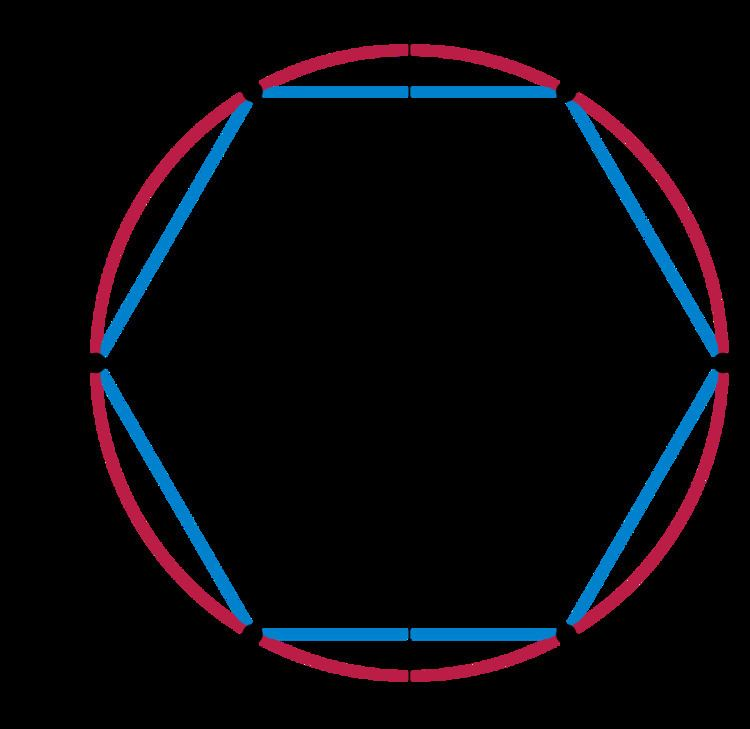# Infinite group

Updated on
Covid-19In group theory, an area of mathematics, an infinite group is a group, of which the underlying set contains an infinite number of elements.

## Examples

• (R, +)
• Infinite Lie groups
• Infinite General linear groups